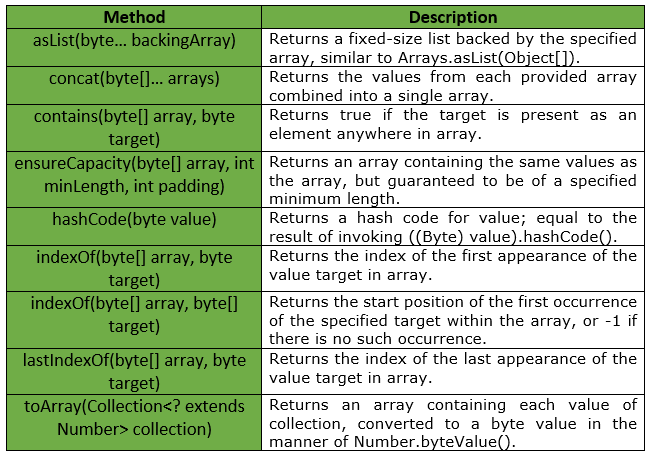# Bytes Class | Guava | Java

Bytes is a utility class for primitive type byte. It provides Static utility methods pertaining to byte primitives, that are not already found in either Byte or Arrays and interpret bytes as neither signed nor unsigned. The methods which specifically treat bytes as signed or unsigned are found in SignedBytes and UnsignedBytes.

Declaration :

```@GwtCompatible(emulated=true)
public final class Bytes
extends Object
```

Below table shows the methods provided by Guava Bytes Class :Exceptions :

• ensureCapacity : IllegalArgumentException if minLength or padding is negative.
• toArray : NullPointerException if collection or any of its elements is null.

Below given are some examples showing the implementation of Guava Bytes Class methods :
Example 1 :

 `// Java code to show implementation ` `// of Guava Bytes.asList() method ` ` `  `import` `com.google.common.primitives.Bytes; ` `import` `java.util.*; ` ` `  `class` `GFG { ` `    ``// Driver method ` `    ``public` `static` `void` `main(String[] args) ` `    ``{ ` `        ``byte` `arr[] = { ``3``, ``4``, ``5``, ``6``, ``7` `}; ` ` `  `        ``// Using Bytes.asList() method which convert ` `        ``// array of primitives to array of objects ` `        ``List myList = Bytes.asList(arr); ` ` `  `        ``// Displaying the elements ` `        ``System.out.println(myList); ` `    ``} ` `} `

Output :

```[3, 4, 5, 6, 7]
```

Example 2 :

 `// Java code to show implementation ` `// of Guava Bytes.indexOf() method ` ` `  `import` `com.google.common.primitives.Bytes; ` `import` `java.util.*; ` ` `  `class` `GFG { ` `    ``// Driver method ` `    ``public` `static` `void` `main(String[] args) ` `    ``{ ` `        ``byte``[] arr = { ``3``, ``4``, ``5``, ``6``, ``7` `}; ` ` `  `        ``// Displaying the index for ` `        ``// first occurrence of given target ` `        ``System.out.println(Bytes.indexOf(arr, (``byte``)``5``)); ` `    ``} ` `} `

Output :

```2
```

Example 3 :

 `// Java code to show implementation ` `// of Guava Bytes.concat() method ` ` `  `import` `com.google.common.primitives.Bytes; ` `import` `java.util.*; ` ` `  `class` `GFG { ` `    ``// Driver method ` `    ``public` `static` `void` `main(String[] args) ` `    ``{ ` `        ``byte``[] arr1 = { ``3``, ``4``, ``5` `}; ` `        ``byte``[] arr2 = { ``6``, ``7` `}; ` ` `  `        ``// Using Bytes.concat() method which ` `        ``// combines arrays from specified ` `        ``// arrays into a single array ` `        ``byte``[] arr = Bytes.concat(arr1, arr2); ` ` `  `        ``// Displaying the elements ` `        ``System.out.println(Arrays.toString(arr)); ` `    ``} ` `} `

Output :

```[3, 4, 5, 6, 7]
```

Example 4 :

 `// Java code to show implementation ` `// of Guava Bytes.contains() method ` ` `  `import` `com.google.common.primitives.Bytes; ` ` `  `class` `GFG { ` `    ``// Driver method ` `    ``public` `static` `void` `main(String[] args) ` `    ``{ ` `        ``byte``[] arr = { ``3``, ``4``, ``5``, ``6``, ``7` `}; ` ` `  `        ``// Using Bytes.contains() method which ` `        ``// checks if element is present in array ` `        ``// or not ` `        ``System.out.println(Bytes.contains(arr, (``byte``)``8``)); ` `        ``System.out.println(Bytes.contains(arr, (``byte``)``7``)); ` `    ``} ` `} `

output :

```false
true
```

Example 5 :

 `// Java code to show implementation ` `// of Guava Bytes.lastIndexOf() method ` ` `  `import` `com.google.common.primitives.Bytes; ` ` `  `class` `GFG { ` `    ``// Driver method ` `    ``public` `static` `void` `main(String[] args) ` `    ``{ ` `        ``byte``[] arr = { ``3``, ``4``, ``5``, ``6``, ``7``, ``5``, ``5` `}; ` ` `  `        ``// Using Bytes.lastIndexOf() method ` `        ``// to get last occurrence of given target ` `        ``System.out.println(Bytes.lastIndexOf(arr, (``byte``)``5``)); ` `    ``} ` `} `

Output :

```6
```

Example 6 :

 `// Java code to show implementation ` `// of Guava Bytes.lastIndexOf() method ` ` `  `import` `com.google.common.primitives.Bytes; ` ` `  `class` `GFG { ` `    ``// Driver method ` `    ``public` `static` `void` `main(String[] args) ` `    ``{ ` `        ``byte``[] arr = { ``3``, ``4``, ``5``, ``6``, ``7``, ``5``, ``5` `}; ` ` `  `        ``// Using Bytes.lastIndexOf() method ` `        ``// to get last occurrence of given target ` `        ``// here target i.e, 9 is not present in ` `        ``// array arr, so -1 will be returned ` `        ``System.out.println(Bytes.lastIndexOf(arr, (``byte``)``9``)); ` `    ``} ` `} `

Output :

```-1
```My Personal Notes arrow_drop_upCheck out this Author's contributed articles.

If you like GeeksforGeeks and would like to contribute, you can also write an article using contribute.geeksforgeeks.org or mail your article to contribute@geeksforgeeks.org. See your article appearing on the GeeksforGeeks main page and help other Geeks.

Please Improve this article if you find anything incorrect by clicking on the "Improve Article" button below.

Improved By : nidhi_biet

Article Tags :
Practice Tags :

Be the First to upvote.

Please write to us at contribute@geeksforgeeks.org to report any issue with the above content.